# BFS in C++: Breadth-First Search Algorithm & Program

BFS is a popular algorithm used to traverse graphs and trees. It is a systematic approach that explores all the vertices at the same level before moving on to the next level. In this article, we will discuss Breadth First Search, how it works, and its implementation in C++. So, let's get started!

## What is Breadth First Search?

BFS is a graph traversal algorithm that starts at the root node and visits all the nodes at the current level before moving on to the next level. BFS follows the First-In-First-Out (FIFO) rule, which means that the first node visited will be the first node to be explored. It is used for searching and finding the shortest path in unweighted graphs.

The algorithm uses a queue data structure to keep track of the nodes that have been visited and the ones that need to be visited.

You can also use it to perform a topological sort of directed acyclic graph (DAG), which is a linear ordering of the nodes that respect the direction of the edges. BFS can detect cycles in a graph as well.

### BFS Algorithm

BFS can be implemented using a queue data structure. The algorithm starts at the root node and adds it to the queue. It then explores all the nodes at the current level by removing the first node from the queue and adding its neighbors to the queue. The algorithm continues this process until all the nodes have been explored.

Here is a step-by-step algorithm for Breadth First Search:

1. Create a queue Q and mark the starting node as visited.
2. Add the starting node to the queue Q.
3. While the queue Q is not empty, do the following:
1. Remove the first node from the queue Q.
2. Explore all the neighbors of the current node.
3. For each unvisited neighbor, mark it as visited and add it to the queue Q.

BFS is a simple yet powerful algorithm for traversing graphs and trees. It is widely used in computer science, including in web crawling, social network analysis, and shortest path finding. The algorithm is easy to implement using a queue data structure and is efficient in finding the shortest path in an unweighted graph.

Let's take a simple example to understand the Breadth-First Search (BFS) algorithm. Consider the following graph: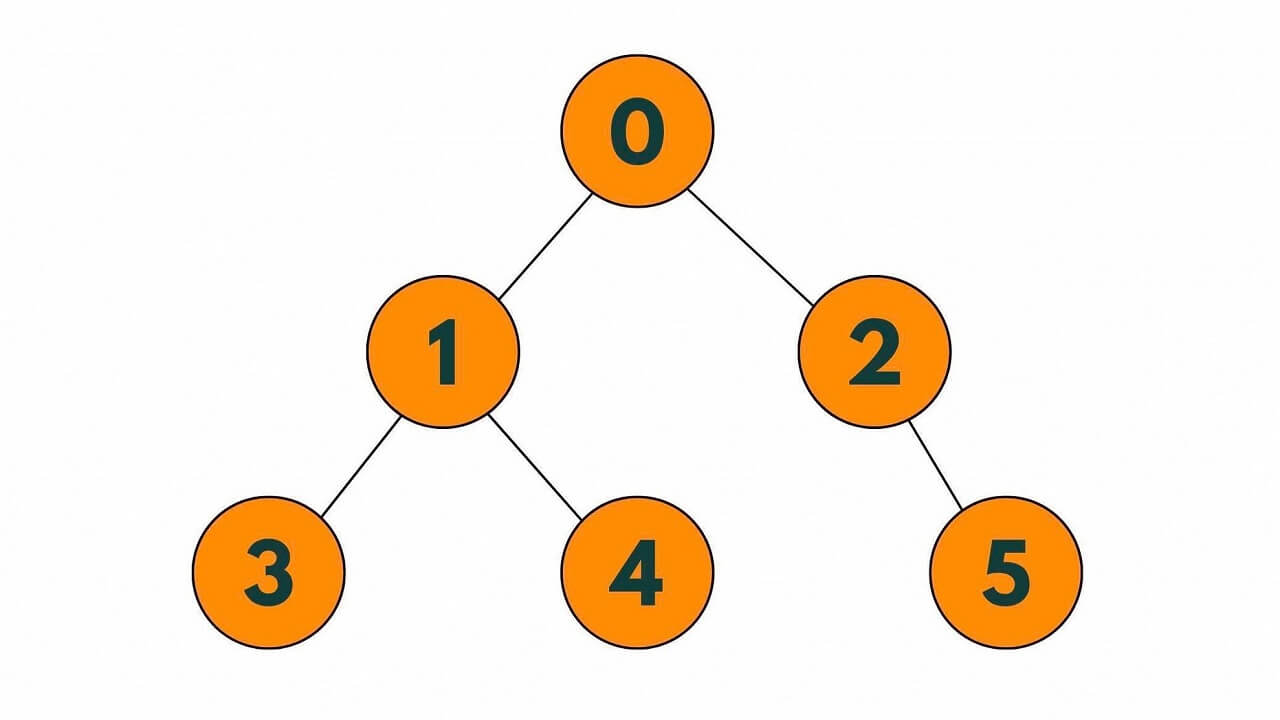To perform a BFS traversal of this graph, we start at node 0 and explore all the nodes at the current level before moving on to the next level. We use a queue to keep track of the nodes that we need to visit next.

Step 1: Start at node 0 and mark it as visited.

Step 2: Add node 0 to the queue.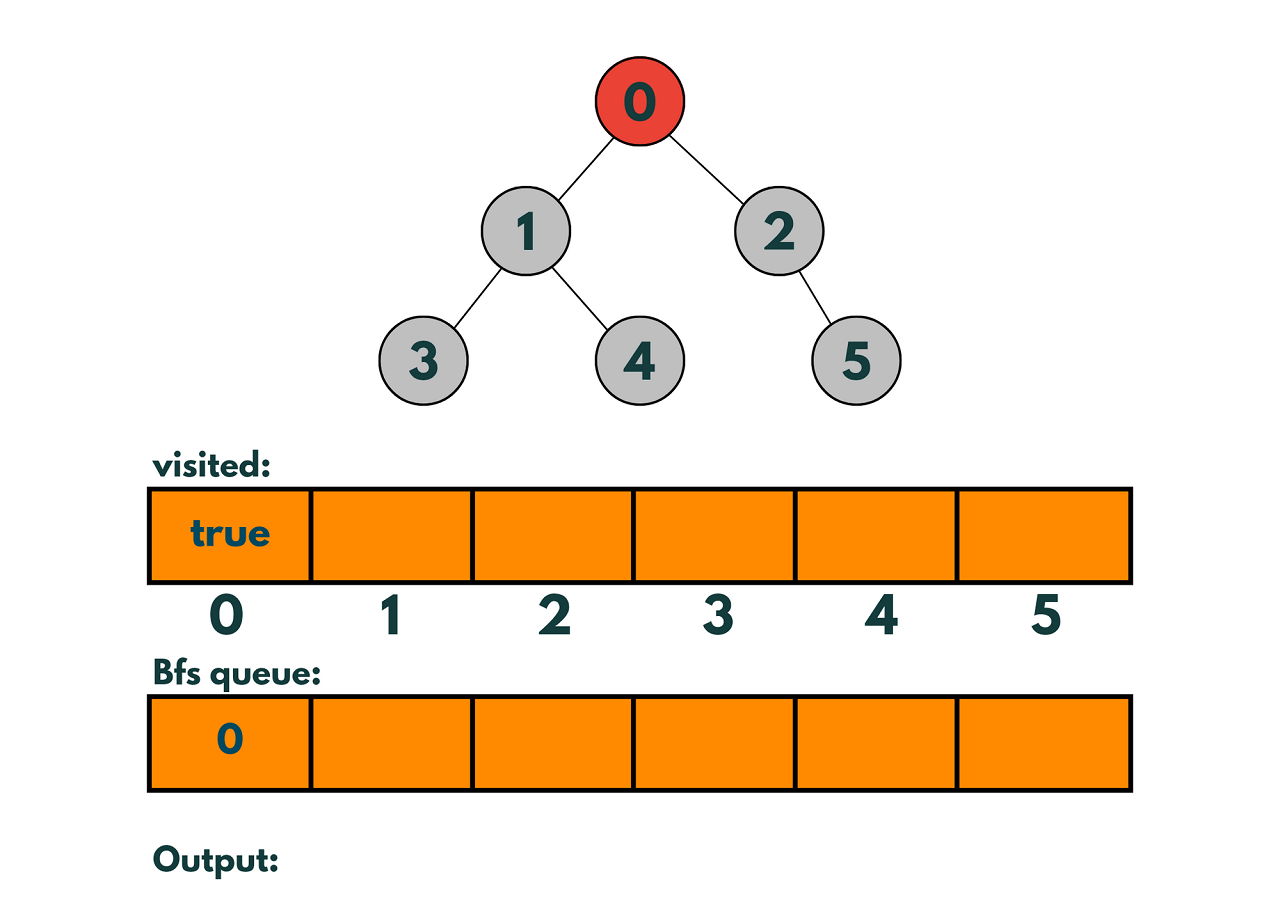Step 3: Remove node 0 from the queue and explore its neighbors: nodes 1 and 2.

Step 4: Mark nodes 1 and 2 as visited and add them to the queue.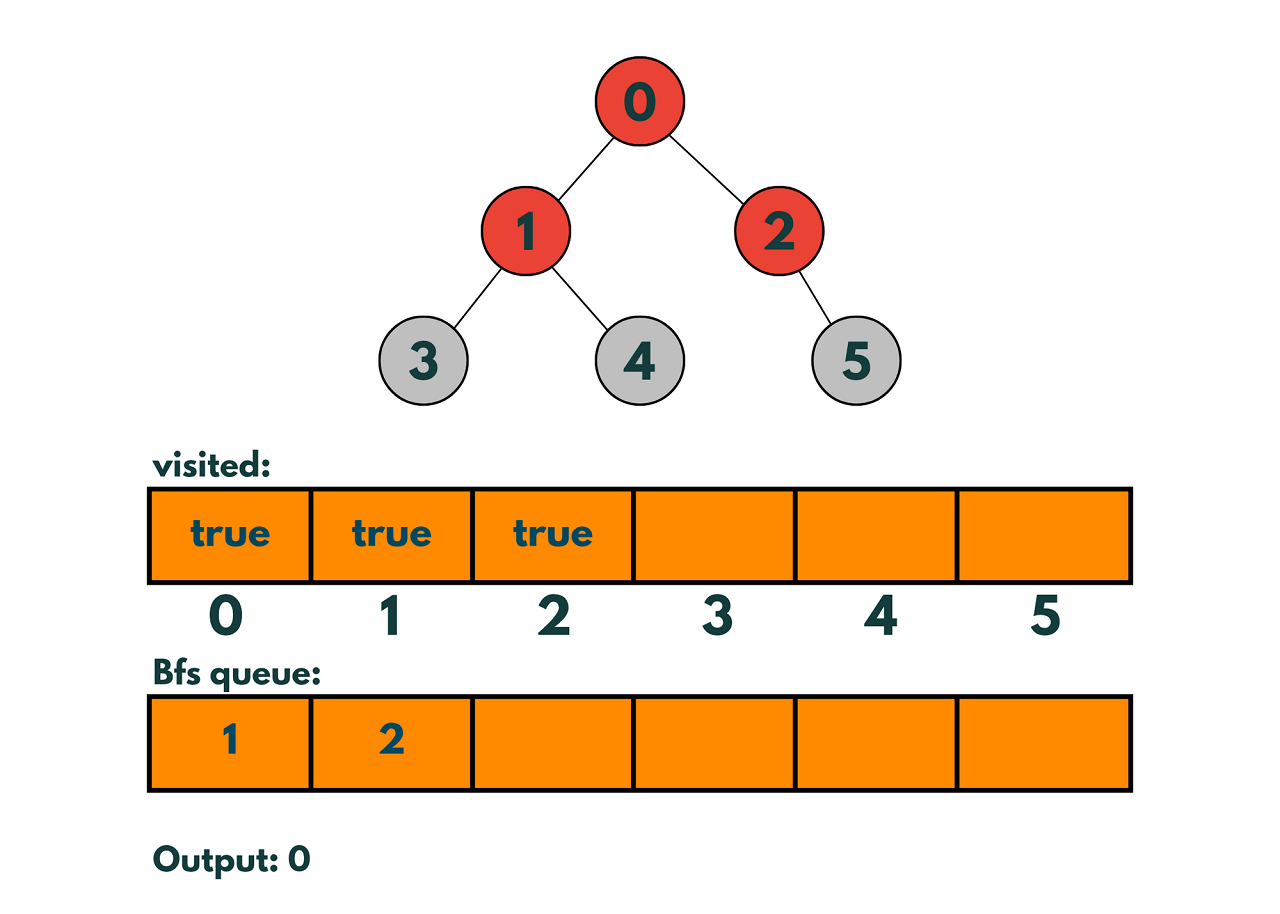Step 5: Remove node 1 from the queue and explore its neighbors: nodes 3 and 4.

Step 6: Mark nodes 3 and 4 as visited and add them to the queue.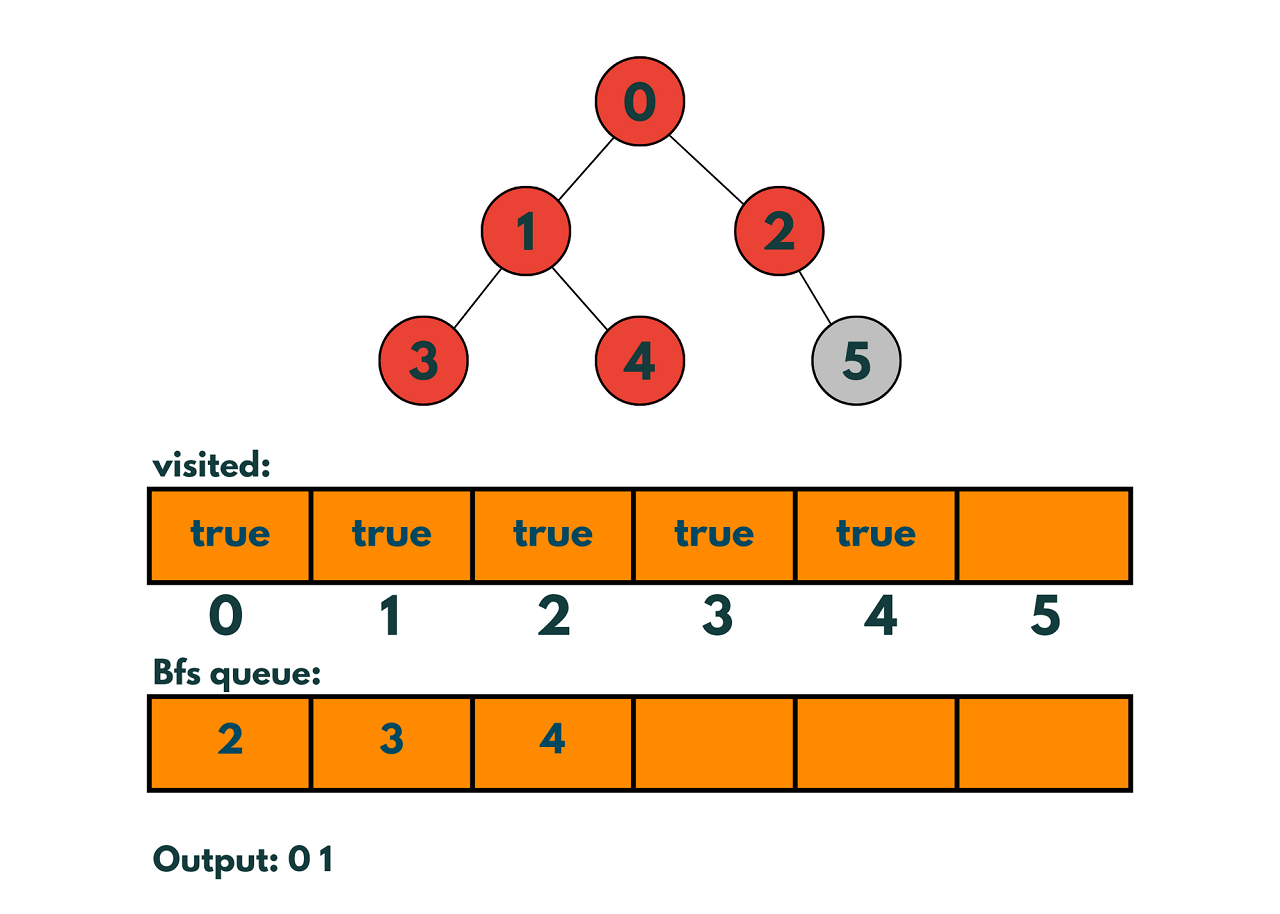Step 7: Remove node 2 from the queue and explore its neighbor: node 5.

Step 8: Mark node 5 as visited and add it to the queue.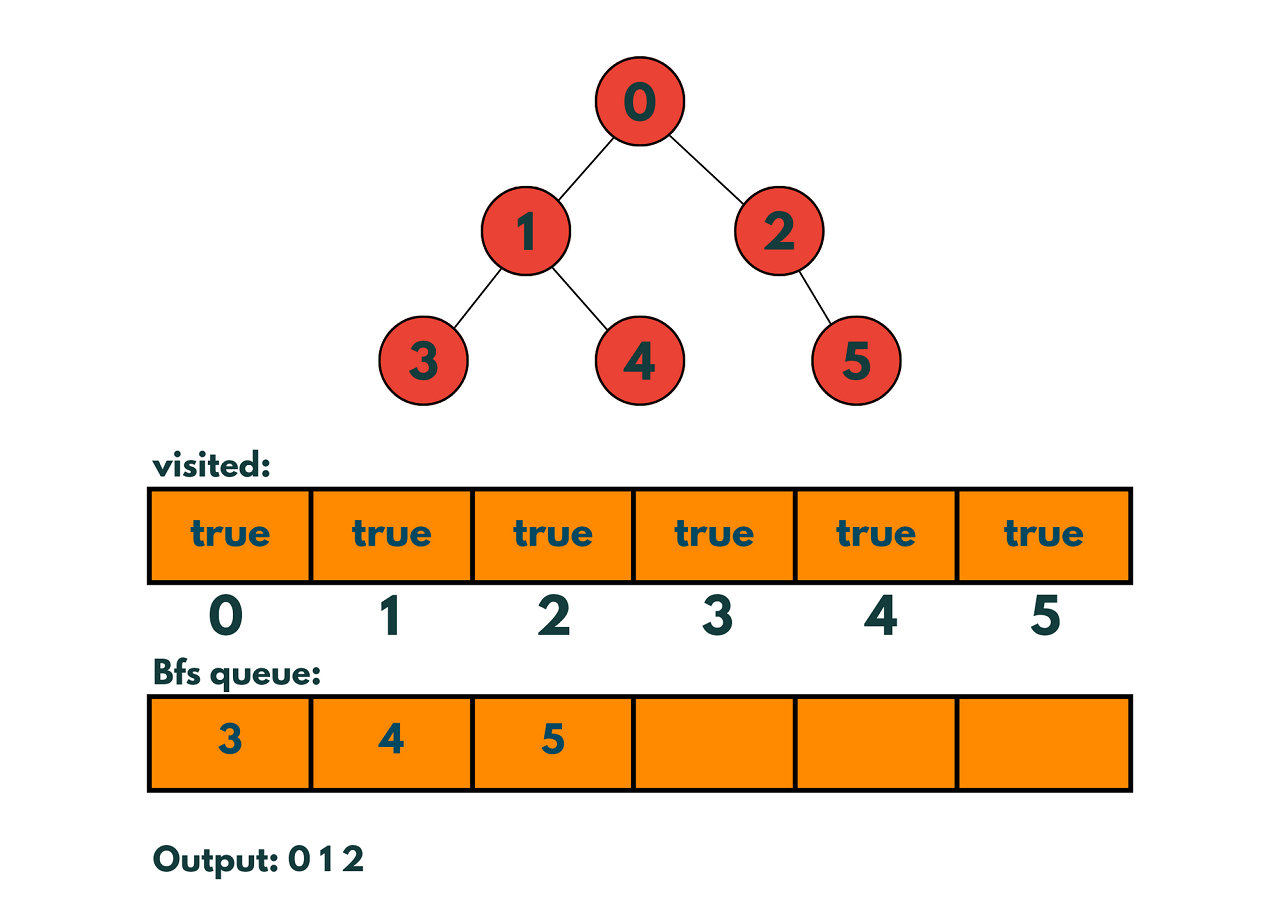Since nodes 3,4 and 5 do not have child nodes we pop them from the queue without anything. The queue is now empty, and all the nodes in the graph have been visited.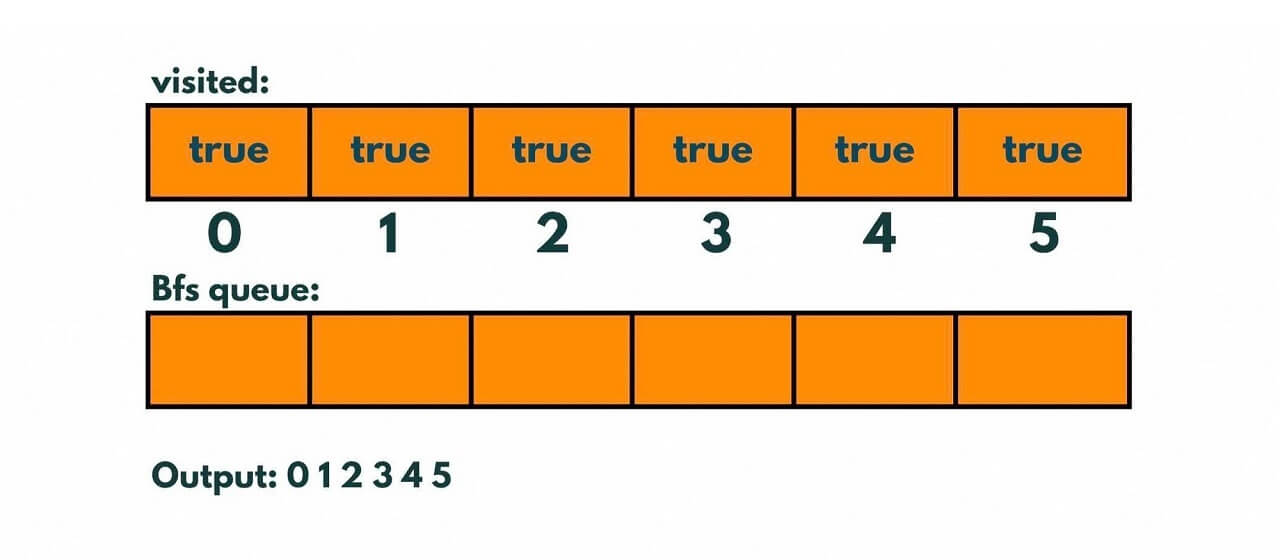The order in which the nodes are visited is 0, 1, 2, 3, 4, 5. This is the BFS traversal of the graph starting at node 0.

To represent the visited nodes, we can use an array with a boolean value for each node. Initially, all the values are set to false, indicating that none of the nodes have been visited. When we visit a node, we set its corresponding value in the array to true.

In our example, the visited array would look like this after the BFS traversal:

```visited = [true, true, true, true, true, true]
```

This indicates that all the nodes in the graph have been visited.

### C++ Program for Breadth-First Search

Here is the complete C++ Program to implement the BFS algorithm:

```#include
#include
#include

using namespace std;

vector<int> bfs(vector<vector<int>>& graph, int start) {
vector<bool> visited(graph.size(), false);
queue<int> q;
vector<int> traversal;

visited[start] = true;
q.push(start);

while (!q.empty()) {
int current = q.front();
q.pop();
traversal.push_back(current);

for (int neighbor : graph[current]) {
if (!visited[neighbor]) {
visited[neighbor] = true;
q.push(neighbor);
}
}
}

return traversal;
}

int main() {
vector<vector<int>> graph = {{1, 2},
{0, 3, 4},
{0, 5},
{1},
{1},
{2}};

vector<int> traversal = bfs(graph, 0);

for (int node : traversal) {
cout << node << " ";
}

return 0;
}
```

Output:

```0 1 2 3 4 5
```

In this example, the BFS function takes a graph represented as an adjacency list and a start node as input. It uses a queue to keep track of the nodes to visit and a visited array to keep track of the nodes that have already been visited. The function returns a vector containing the order in which the nodes were visited during the BFS traversal.

In the main function, we define a graph using an adjacency list and call the BFS function with node 0 as the starting node. Finally, we print the traversal sequence to the console.

Note that this implementation assumes that the graph is connected. If the graph is not connected, we need to call the BFS function multiple times, starting from each unvisited node, to visit all the nodes in the graph.

### Space and Time Complexity

The time and space complexity of BFS (Breadth-First Search) depends on the size and structure of the graph being traversed.

The time complexity of BFS is O(V+E), where V is the number of vertices and E is the number of edges in the graph. This is because BFS visits each vertex and edge at most once. In the worst-case scenario, BFS needs to visit all the vertices and edges of the graph to find the shortest path between two nodes. Therefore, the time complexity of BFS is linear in the size of the graph.

The space complexity of BFS is O(V), where V is the number of vertices in the graph. This is because BFS needs to keep track of the visited nodes and the nodes to be visited in a queue. In the worst-case scenario, BFS needs to store all the vertices in the queue to find the shortest path between two nodes.

As we said before, BFS is an efficient algorithm for finding the shortest path in an unweighted graph.

One of the biggest advantages of Breadth-First Search is its Completeness. It is guaranteed to find a solution if one exists. It is also memory-efficient because it does not require a lot of memory because it only needs to store the nodes at the current level.

But there is also its high time complexity of O(V+E), which is big limited for larger graphs. Also, if the graph contains a cycle, BFS can get stuck in an infinite loop, visiting the same nodes over and over again.

In such cases, other algorithms like Dijkstra's algorithm in C++ may be more suitable.

## Conclusion

Overall, BFS in C++ is a versatile algorithm that can be applied to a wide range of problems that involve graph traversal and search. Now you can easily implement Breadth-First Search Algorithm. It is used in the practical world for Google web crawling, social networking websites, and puzzle solving (as Rubik's cube) by searching for the shortest sequence of moves.

### FavTutor - 24x7 Live Coding Help from Expert Tutors!